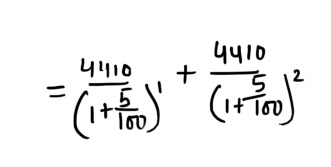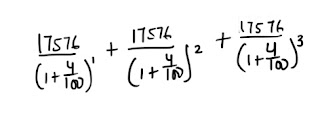# Installments : 8 Questions Repeated in the Exams

From past 4 years I am analyzing the paper pattern of various competitive exams, in every exam you will find at least 1 question from this chapter. Few questions are repeated every year. Today I am sharing all those questions.#### Ques 1.

An electric iron is sold for Rs 110 cash or Rs 50 cash down payment followed by Rs 62 after a month. Find the rate of interest charged under the installment plan.
Ans 1.  Present worth of the amount to be  paid in installment = Rs (110-50) = Rs 60
Let the rate of interest be r% p.a.
After a month the worth of Rs 60 would be : Rs ( 60 + 20 xr /100 x 1/12)
But 60 + r/20 = 62
∴ r = ( 62 - 60 ) 20 = 40 % p.a.

#### Ques 2.

A loan of Rs 2550 is to be paid back in two equal half - yearly installments . How much is each installment  if the interest is compounded half - yearly at 8% p.a.?
Ans 2. Present  worth  of loan = Rs 2550
Let each installment be Rs x.#### Ques 3.

Kusum  borrowed money and returned  it in 3 equal  quarterly installments of Rs.4630.50 each . What sum had she borrowed if the rate of interest was 20% p.a. compounded quarterly ? Find also the total interest charged .
Ans 3. Using the concept of present  worth  we have :Total value of the three installment = Rs (4630.50 x 3) = Rs 13891.50
∴ Total interest charged = Rs(13891.50 - 12610) = 1281.50.

#### Ques 4.

A steel container  is sold at Rs  120 cash or Rs 25 as cash down payment and Rs.25 a month for 4 months .The rate of interest per annum charged under the installment plan is .
(a) 26.09%
(b) 24.09%
(c) 20 %
(d) 23.25%
Ans 4. 26.09%
Hint :  Present  worth of the balance  amount to be paid = Rs (120-25) = Rs  95
Worth of Rs  95 after  4 months =#### Ques 5.

A sum of Rs 7500 is to be paid back in three equal annual installments .How much is each installment if the interest is compounded annually at 4% p.a.?
(a) Rs. 1000.05
(b) Rs  981.65
(c) Rs 163.58
(d) Rs 2701 .61
Ans 5. 2701.61
Hint : 7500 =#### Ques 6.

A man borrows  money on compound interest and return  it in  two equal  half - yearly installments of Rs 4410  each. Find the interest  charged if the rate of interest  is 10 % p.a. compounded half - yearly.
(a) Rs 600
(b) Rs 720
(c) Rs 620
(d) Rs 660
Ans 6. RS  620
Hint : Sum  borrowed#### Ques 7.

Sunder borrowed money  and returned it in 3 equal quarterly installments of Rs. 17576 each . What  sum had he  borrowed if the rate of  interest was 16% p.a. compounded quarterly ?
(a) Rs 50000
(b) Rs 48786
(c) Rs 48775
(d) None of these
Ans 7.  48775
Hint : Required sum =#### Ques 8.

A loan  of Rs 8400 is to be paid  in two equal half - yearly installment the interest  being charged at 10% per annum compounded half - yearly . Find each installment( approx ).
(a) Rs 4450
(b) Rs 4400
(c) Rs 4850
(d) Rs 4500

Ans 8. 4500

#### Free Notes with Question Bank